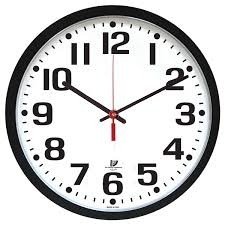# Math homework

It took Jose two-thirds of an hour to complete his math homework on Monday, three-fourths of an hour on Tuesday, and any two-fifths of an hour on Wednesday. How many hours did it take Jose to complete his homework altogether?

Result

t = 1:49 h### Step-by-step explanation:Did you find an error or inaccuracy? Feel free to write us. Thank you!

Tips for related online calculators
Need help calculating sum, simplifying, or multiplying fractions? Try our fraction calculator.
Do you want to convert time units like minutes to seconds?